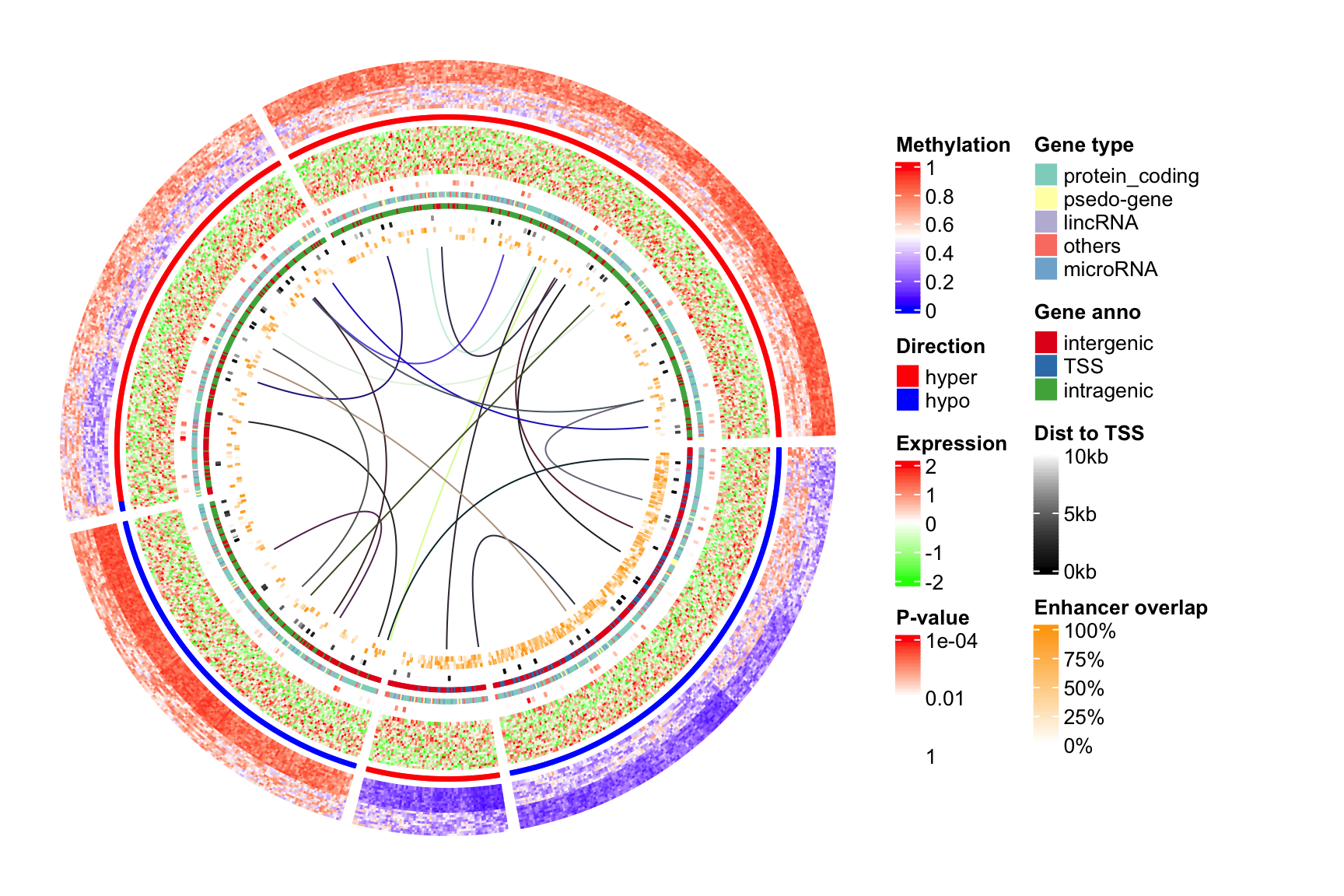# Changes in circlize 0.4.10

circlize 0.4.10 is now on CRAN. Besides the bug fixes, following are the user-visible changes/features.

``````library(circlize)
set.seed(123)``````

## Add circos.barplot(), circos.boxplot() and circos.violin()

`circos.barplot()`, `circos.boxplot()` and `circos.violin()` are used inside the `panel.fun`. Please note the values on x-axes are the integer indices of bars, boxes or violins for which `xlim` should be properly set in `circos.initialize()`.

### Barplots

For circular barplots, you can either specify a vector which generates a “normal” barplot, or a matrix which generates a stacked barplot.

``````par(mfrow = c(1, 2))
circos.initialize(fa = letters[1:4], xlim = c(0, 10))
circos.track(ylim = c(0, 1), panel.fun = function(x, y) {
value = runif(10)
circos.barplot(value, 1:10 - 0.5, col = 1:10)
})
circos.track(ylim = c(-1, 1), panel.fun = function(x, y) {
value = runif(10, min = -1, max = 1)
circos.barplot(value, 1:10 - 0.5, col = ifelse(value > 0, 2, 3))
})
circos.clear()

circos.initialize(fa = letters[1:4], xlim = c(0, 10))
circos.track(ylim = c(0, 4), panel.fun = function(x, y) {
value = matrix(runif(10*4), ncol = 4)
circos.barplot(value, 1:10 - 0.5, col = 2:5)
})``````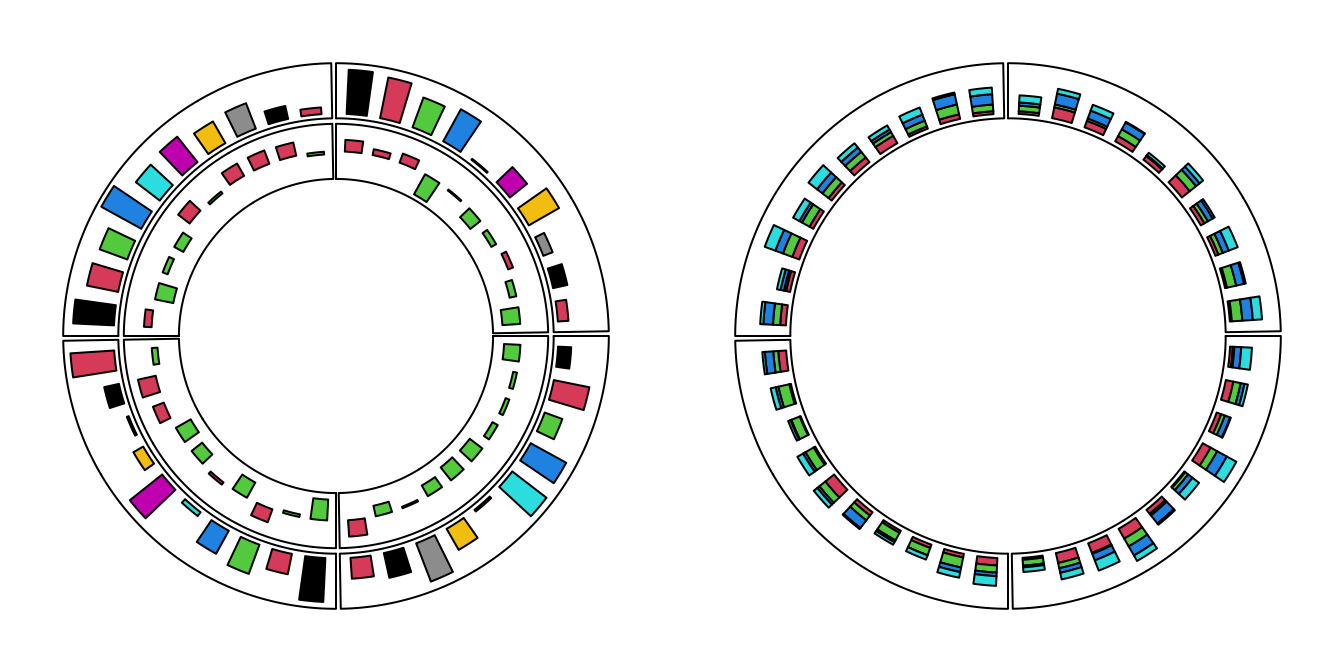``circos.clear()``

### Boxplot

For circular boxplots, the boxes can be drawn one-by-one by providing a vector for each box, or drawn in batch with a list/matrix as input.

``````par(mfrow = c(1, 2))
circos.initialize(fa = letters[1:4], xlim = c(0, 10))
circos.track(ylim = c(0, 1), panel.fun = function(x, y) {
for(pos in seq(0.5, 9.5, by = 1)) {
value = runif(10)
circos.boxplot(value, pos)
}
})
circos.clear()

circos.initialize(fa = letters[1:4], xlim = c(0, 10))
circos.track(ylim = c(0, 1), panel.fun = function(x, y) {
value = replicate(runif(10), n = 10, simplify = FALSE)
circos.boxplot(value, 1:10 - 0.5, col = 1:10)
})``````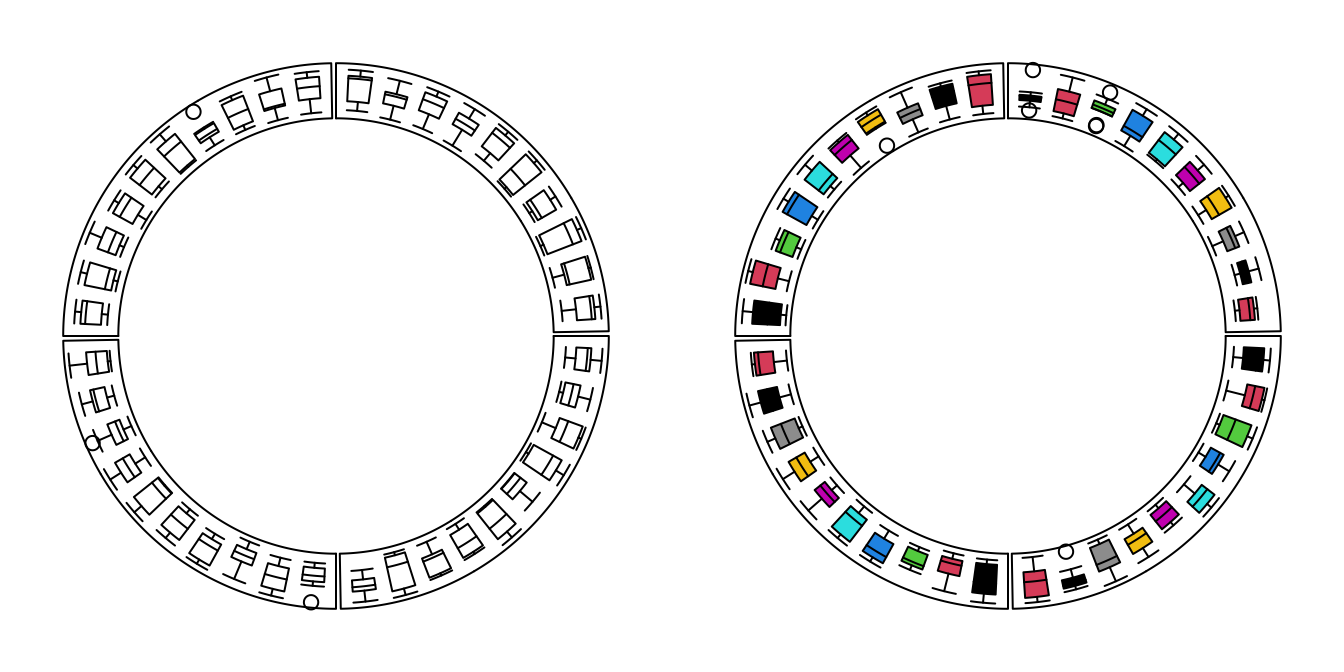``circos.clear()``

### Violin plots

For circular violin plots, the violins can be drawn one-by-one by providing a vector for each violin, or drawn in batch with a list/matrix as input.

Please note, to make it comparable between violins, `max_density` argument should be set.

``````par(mfrow = c(1, 2))
circos.initialize(fa = letters[1:4], xlim = c(0, 10))
circos.track(ylim = c(0, 1), panel.fun = function(x, y) {
for(pos in seq(0.5, 9.5, by = 1)) {
value = runif(10)
circos.violin(value, pos)
}
})
circos.clear()

circos.initialize(fa = letters[1:4], xlim = c(0, 10))
circos.track(ylim = c(0, 1), panel.fun = function(x, y) {
value = replicate(runif(10), n = 10, simplify = FALSE)
circos.violin(value, 1:10 - 0.5, col = 1:10)
})``````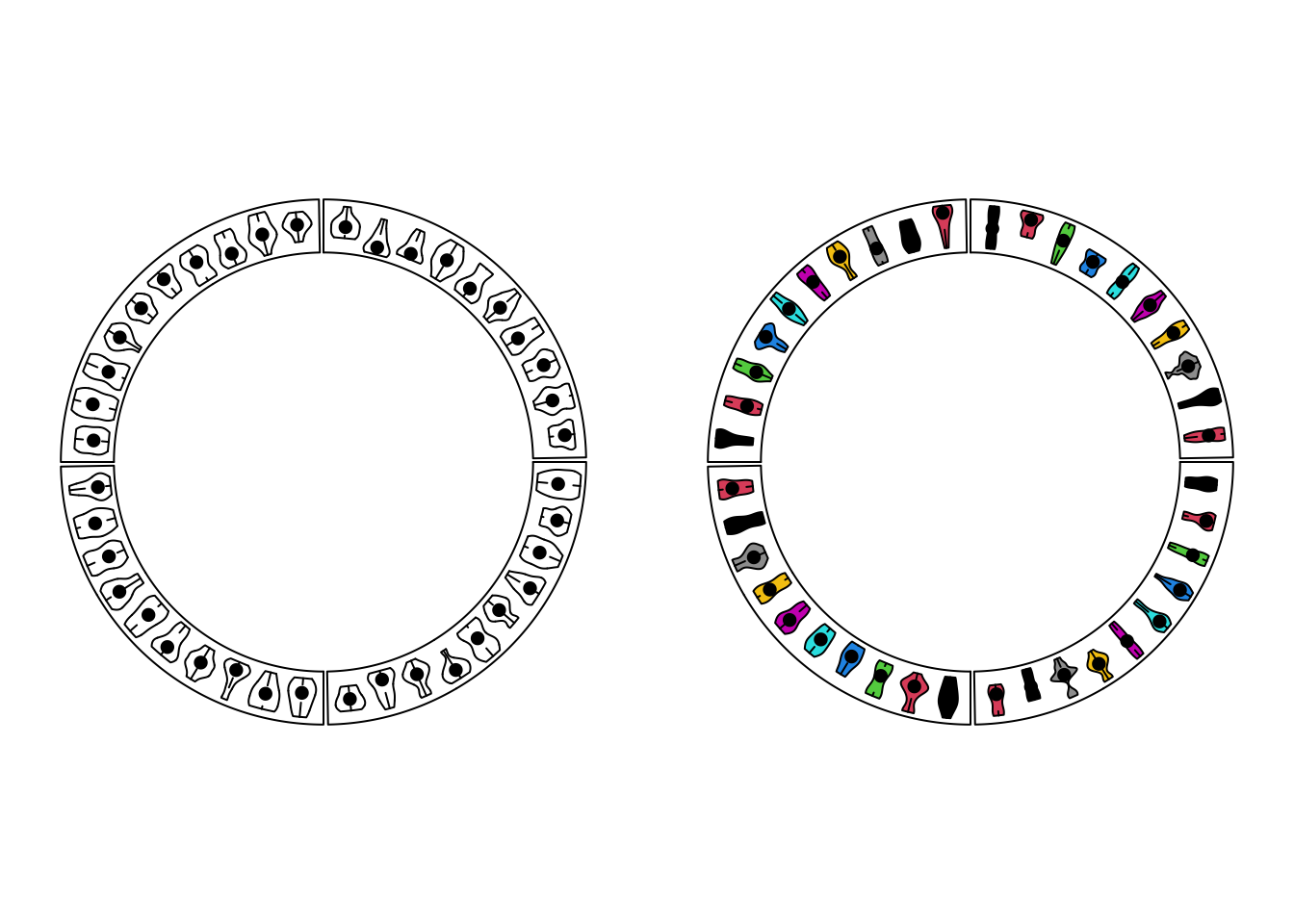``circos.clear()``

## Physical units

In the older versions, the widths/heights/lengths are specified by `convert_x()`/`convert_y()`/`convert_height()` functions, however, the function names are not straightforward. Now there are following functions that control the physical size:

• `mm_x()`/`cm_x()`/`inch_x()`: on x-axes in the cells.
• `mm_y()`/`cm_y()`/`inch_y()`: on y-axes in the cells.
• `mm_h()`/`cm_h()`/`inch_h()`: control the height of track and the gap between tracks.

See the following example:

``````fa = letters[1:10]
circos.par(cell.padding = c(0, 0, 0, 0), track.margin = c(0, 0))
circos.initialize(fa, xlim = cbind(rep(0, 10), runif(10, 0.5, 1.5)))
circos.track(ylim = c(0, 1), track.height = mm_h(5),
panel.fun = function(x, y) {
circos.lines(c(0, 0 + mm_x(5)), c(0.5, 0.5), col = "blue")
})
set_track_gap(mm_h(2))
circos.track(ylim = c(0, 1), track.height = cm_h(1),
panel.fun = function(x, y) {
xcenter = get.cell.meta.data("xcenter")
circos.lines(c(xcenter, xcenter), c(0, cm_y(1)), col = "red")
})
set_track_gap(mm_h(5))
circos.track(ylim = c(0, 1), track.height = inch_h(0.5),
panel.fun = function(x, y) {
line_length_on_x = cm_x(1*sqrt(2)/2)
line_length_on_y = cm_y(1*sqrt(2)/2)
circos.lines(c(0, line_length_on_x), c(0, line_length_on_y), col = "orange")
})``````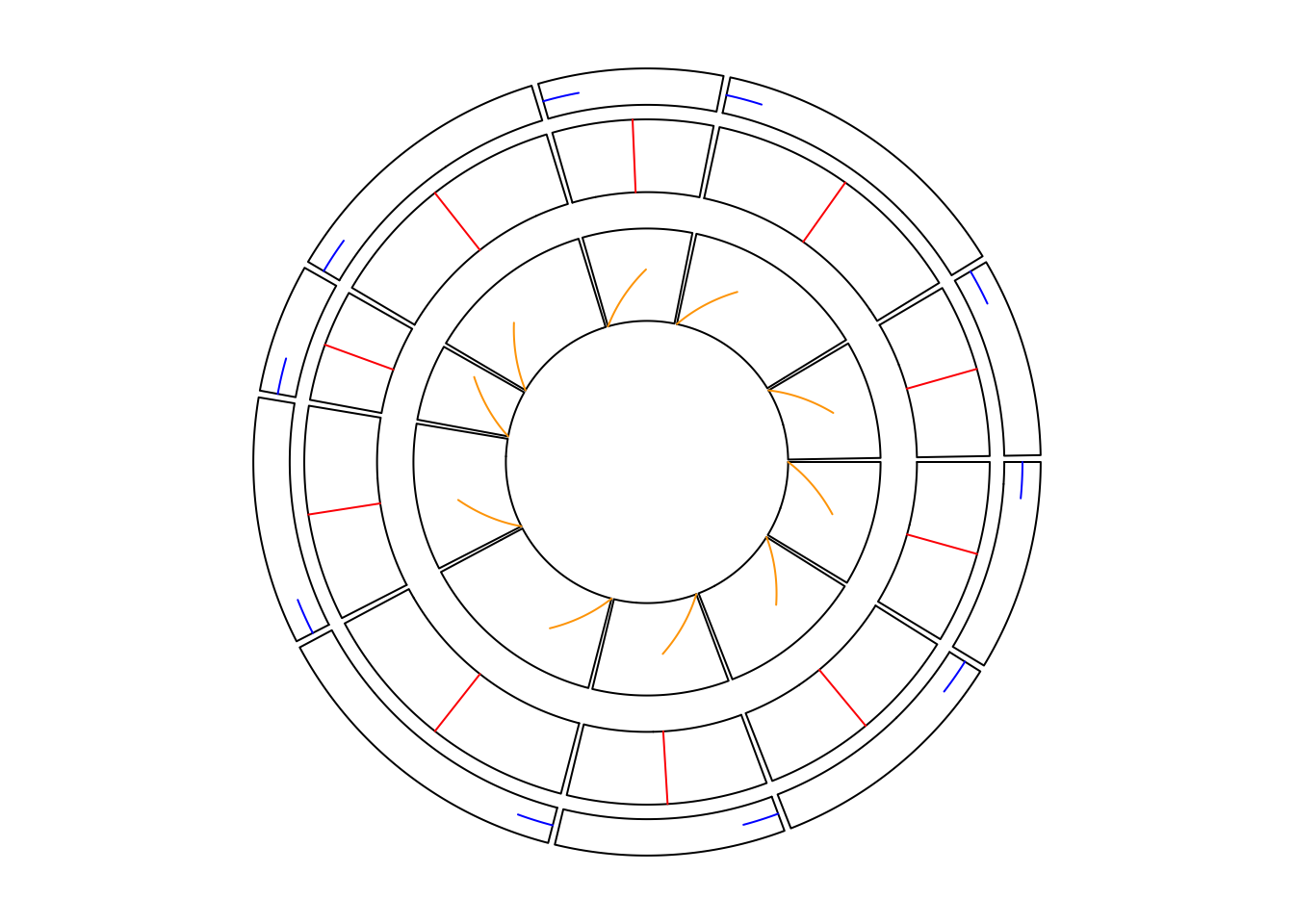``circos.clear()``

## Set gaps between tracks

In the older version, you need to set `track.height` parameter either in `circos.par()` or in `circos.track()` to control the space between tracks. Now there is a new `set_track_gap()` function which simplifies the setting of gaps between two tracks. With the `mm_h()`/`cm_h()`/`inch_h()` functions, it is very easy to set the gaps with physical units.

``````circos.initialize(fa = letters[1:10], xlim = c(0, 1))
circos.track(ylim = c(0, 1))
set_track_gap(mm_h(2)) # 2mm
circos.track(ylim = c(0, 1))
set_track_gap(cm_h(0.5)) # 0.5cm
circos.track(ylim = c(0, 1))``````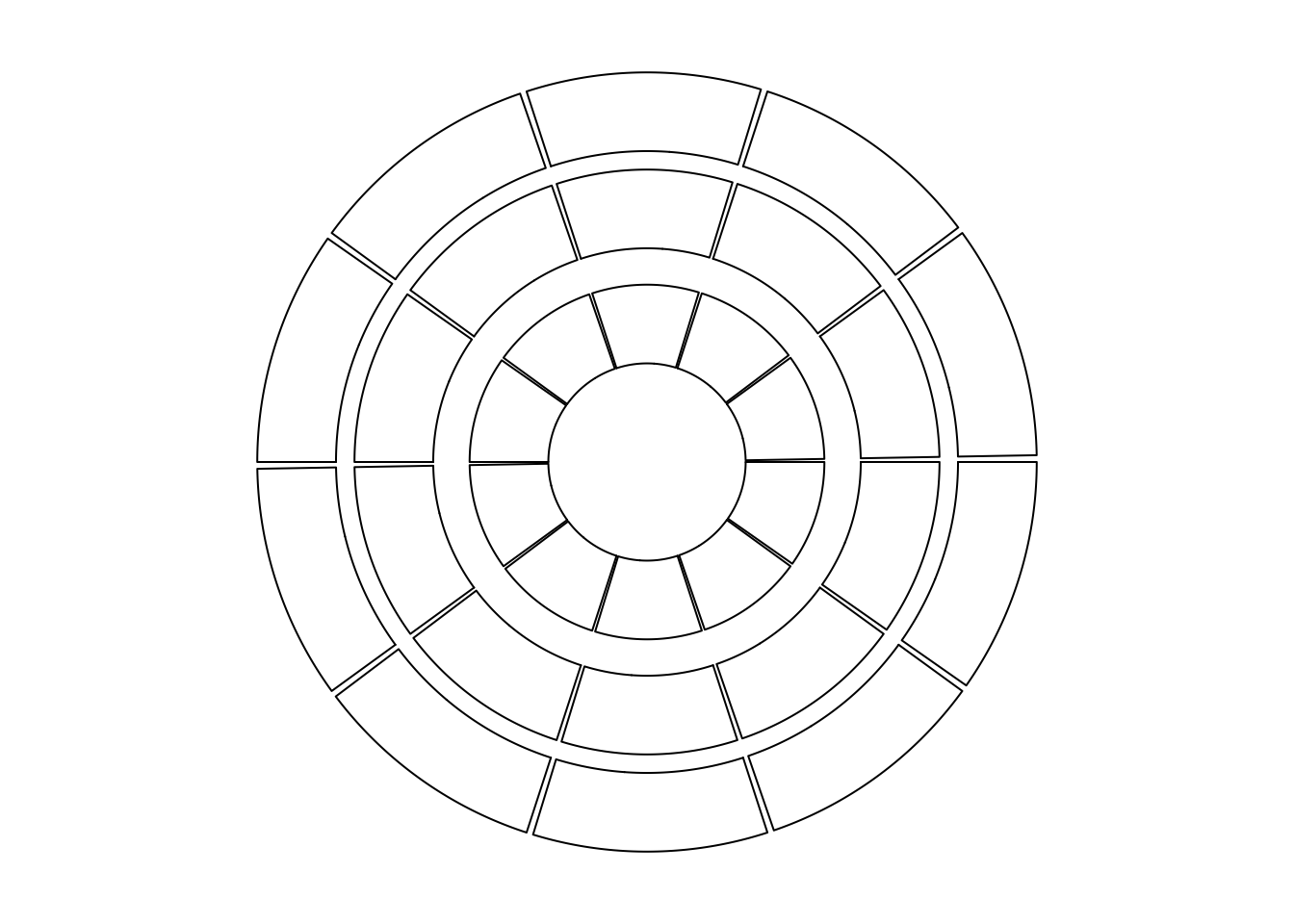``circos.clear()``

## gap.degree with names

`circos.par()` is aimed to be designed to be independent to the number or the order of sectors, however, there is an exception. The `gap.degree` parameter controls the spaces between two neighbouring sectors. When it is set as a scalar, the gap is the same to every two neighbouring sectors and it works fine. It can also be a vector which has the same length as the number of sectors and it can cause problems:

1. When you change the order of the sectors, you also need to manually change the order of `gap.degree`.
2. in `chordDiagram()` function, by default the tiny sectors are removed to improve the visualization, which means, the `gap.degree` should be adjusted.
3. Also in `chordDiagram()`, sometimes it is not very straightforward to find out the order of sectors, thus, it is difficult to set a proper `gap.degree`.

Now, from version 0.4.10, the value of `gap.degree` can be set as a named vector where the names are the names of the sectors. In this case, the `gap.degree` vector can be automatically reordered or subsetted according to the sector ordering in the plot.

## Multi-group Chord diagram

Please see https://jokergoo.github.io/2020/06/08/multiple-group-chord-diagram/. An example is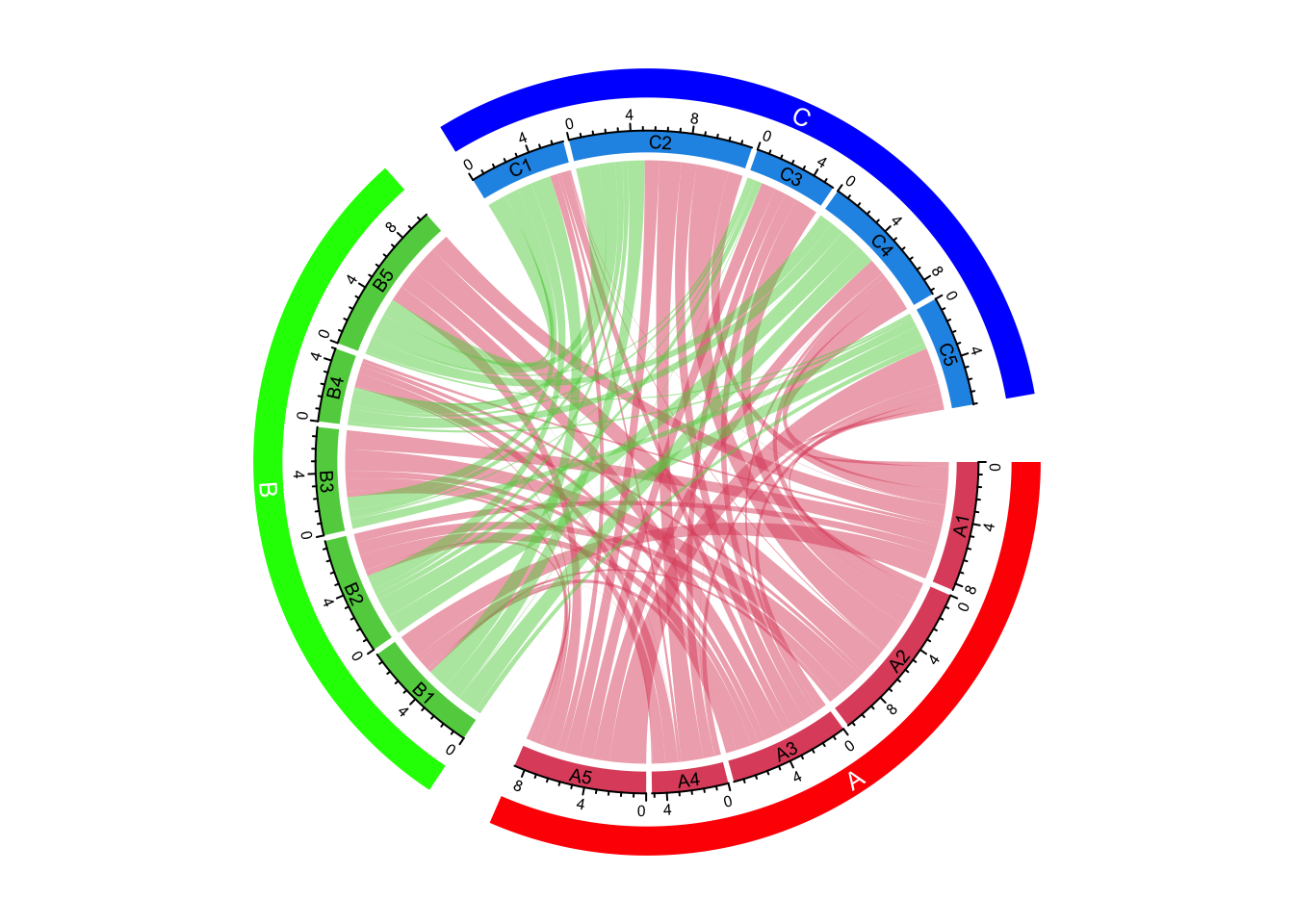## Circular Heatmaps

Please see https://jokergoo.github.io/2020/05/21/make-circular-heatmaps/. An example is